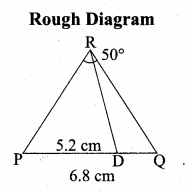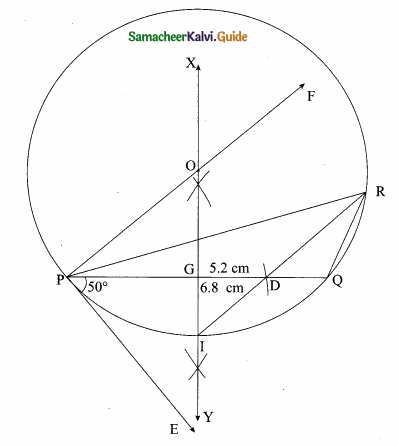Students can download Maths Chapter 4 Geometry Ex 4.2 Questions and Answers, Notes, Samacheer Kalvi 10th Maths Guide Pdf helps you to revise the complete Tamilnadu State Board New Syllabus, helps students complete homework assignments and to score high marks in board exams.

## Tamilnadu Samacheer Kalvi 10th Maths Solutions Chapter 4 Geometry Ex 4.2

Question 1.
In ∆ABC, D and E are points on the sides AB and AC respectively such that DE || BC
(i) If $$\frac { AD }{ DB }$$ = $$\frac { 3 }{ 4 }$$ and AC = 15 cm find AE.
(ii) If AD = 8x – 7 , DB = 5x – 3 , AE = 4x – 3 and EC = 3x – 1, find the value of x.
Solution:
(i) Let AE be x
∴ EC = 15 – x
In ∆ABC we have DE || BC
By Basic proportionality theorem, we have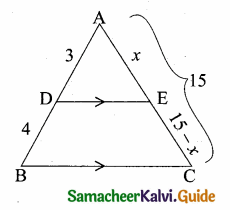$$\frac { AD }{ DB }$$ = $$\frac { AE }{ EC }$$
$$\frac { 3 }{ 4 }$$ = $$\frac { x }{ 15-x }$$
4x = 3 (15 – x)
4x = 45 – 3x
7x = 45 ⇒ x = $$\frac { 45 }{ 7 }$$ = 6.43
The value of x = 6.43(ii) Given AD = 8x – 7; BD = 5x – 3; AE = 4x – 3; EC = 3x – 1
In ∆ABC we have DE || BC
By Basic proportionality theorem
$$\frac { AD }{ DB }$$ = $$\frac { AE }{ EC }$$
$$\frac { 8x-7 }{ 5x-3 }$$ = $$\frac { 4x-3 }{ 3x-1 }$$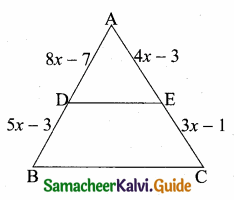(8x – 7) (3x – 1) = (4x – 3) (5x – 3)
24x2 – 8x – 21x + 7 = 20x2 – 12x – 15x + 9
24x2 – 20x2 – 29x + 27x + 7 – 9 = 0
4x2 – 2x – 2 = 0
2x2 – x – 1 = 0 (Divided by 2)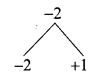2x2 – 2x + x – 1 = 0
2x(x -1) + 1 (x – 1) = 0
(x – 1) (2x + 1) = 0
x – 1 = 0 or 2x + 1 = 0
x = 1 or 2x = -1 ⇒ x = – $$\frac { 1 }{ 2 }$$ (Negative value will be omitted)
The value of x = 1Question 2.
ABCD is a trapezium in which AB || DC and P,Q are points on AD and BC respectively, such that PQ || DC if PD = 18 cm, BQ
Solution:
Join AC intersecting PQ at S.
Let AP be x
∴ AD = x + 18
In the ∆ABC, QS || AB
By basic proportionality theorem.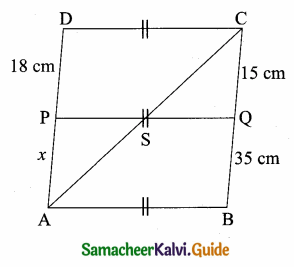$$\frac { AS }{ SC }$$ = $$\frac { BQ }{ QC }$$
$$\frac { AS }{ SC }$$ = $$\frac { 35 }{ 15 }$$ ………(1)
In the ∆ACD; PS || DC
By basic proportionality theorem.
$$\frac { AS }{ SC }$$ = $$\frac { AP }{ PD }$$
$$\frac { AS }{ SC }$$ = $$\frac { x }{ 18 }$$ ………..(2)
From (1) and (2) we get
$$\frac { 35 }{ 15 }$$ = $$\frac { x }{ 18 }$$
15x = 35 × 18 ⇒ x = $$\frac{35 \times 18}{15}$$ = 42
= 42 + 18 = 60
The value of AD = 60 cmQuestion 3.
In ∆ABC, D and E are points on the sides AB and AC respectively. For each of the following cases show that DE || BC.
(i) AB = 12 cm, AD = 8 cm, AE = 12 cm and AC = 18 cm.
(ii) AB = 5.6 cm, AD = 1.4 cm, AC = 7.2 cm and AE = 1.8 cm.
Solution:
(i) Here AB = 12 cm; BD =12 – 8 = 4 cm; AE =12 cm; EC = 18 – 12 = 6 cm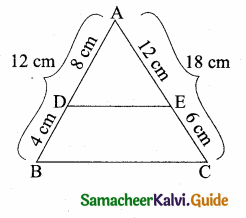∴ $$\frac { AD }{ DB }$$ = $$\frac { 8 }{ 4 }$$ = 2
$$\frac { AE }{ EC }$$ = $$\frac { 12 }{ 6 }$$ = 2
$$\frac { AD }{ DB }$$ = $$\frac { AE }{ EC }$$
By converse of basic proportionality theorem DE || BC

(ii) Here AB = 5.6 cm; AD = 1.4 cm;
= 5.6 – 1.4 = 4.2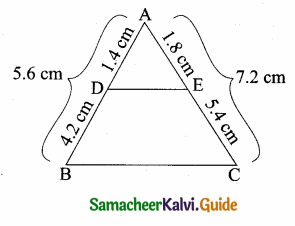AC = 7.2 cm; AE = 1.8 cm
EC = AC – AE
= 7.2 – 1.8
EC = 5.4 cm
$$\frac { AD }{ DB }$$ = $$\frac { 1.4 }{ 4.2 }$$ = $$\frac { 1 }{ 3 }$$
$$\frac { AE }{ EC }$$ = $$\frac { 1.8 }{ 5.4 }$$ = $$\frac { 1 }{ 3 }$$
$$\frac { AE }{ EC }$$ = $$\frac { AD }{ DB }$$
By converse of basic proportionality theorem DE || BCQuestion 4.
In fig. if PQ || BC and BC and PR || CD prove that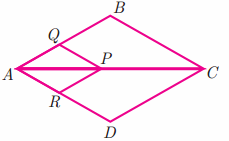(i) $$\frac { AR }{ AD }$$ = $$\frac { AQ }{ AB }$$
(ii) $$\frac { QB }{ AQ }$$ = $$\frac { DR }{ AR }$$
Solution:
(i) In ∆ABC, We have PQ || BC
By basic proportionality theorem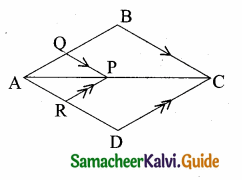$$\frac { AQ }{ AB }$$ = $$\frac { AP }{ AC }$$ ……(1)
In ∆ACD, We have PR || CD
basic proportionality theorem
$$\frac { AP }{ AC }$$ = $$\frac { AR }{ AD }$$ ………..(2)
From (1) and (2) we get
$$\frac { AQ }{ AB }$$ = $$\frac { AR }{ AD }$$ (or) $$\frac { AR }{ AD }$$ = $$\frac { AQ }{ AB }$$

(ii) In ∆ABC, PQ || BC (Given)
By basic proportionality theorem
$$\frac { AP }{ PC }$$ = $$\frac { AQ }{ QB }$$ ………..(1)
In ∆ADC, PR || CD (Given)
By basic proportionality theorem
$$\frac { AP }{ PC }$$ = $$\frac { AR }{ RD }$$ ………(2)
From (1) and (2) we get
$$\frac { AQ }{ QB }$$ = $$\frac { AP }{ RD }$$ (or) $$\frac { QB }{ AQ }$$ = $$\frac { RD }{ AR }$$Question 5.
Rhombus PQRB is inscribed in ∆ABC such that ∠B is one of its angle. P, Q and R lie on AB, AC and BC respectively. If AB = 12 cm and BC = 6 cm, find the sides PQ, RB of the rhombus.
Solution:
Let the side of the rhombus be “x”. Since PQRB is a Rhombus PQ || BC
By basic proportionality theorem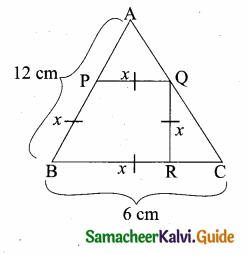$$\frac { AP }{ AB }$$ = $$\frac { PQ }{ BC }$$ ⇒ $$\frac { 12-x }{ BC }$$ = $$\frac { x }{ 6 }$$
12x = 6 (12 – x)
12x = 72 – 6x
12x + 6x = 72
18x = 72 ⇒ x = $$\frac { 72 }{ 18 }$$ = 4
Side of a rhombus = 4 cm
PQ = RB = 4 cmQuestion 6.
In trapezium ABCD, AB || DC , E and F are points on non-parallel sides AD and BC respectively, such that EF || AB.
Show that = $$\frac { AE }{ ED }$$ = $$\frac { BF }{ FC }$$
Solution:
Given: ABCD is a trapezium AB || DC
E and F are the points on the side AD and BC
EF || AB
To Prove: $$\frac { AE }{ ED }$$ = $$\frac { BF }{ FC }$$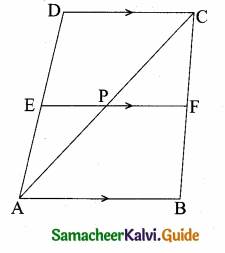Construction: Join AC intersecting AC at P
Proof:
In ∆ABC, PF || AB (Given)
By basic proportionality theorem
$$\frac { AP }{ PC }$$ = $$\frac { BF }{ FC }$$ ………..(1)
In the ∆ACD, PE || CD (Given)
By basic Proportionality theorem
$$\frac { AP }{ PC }$$ = $$\frac { AE }{ ED }$$ …………..(2)
From (1) and (2) we get
$$\frac { AE }{ ED }$$ = $$\frac { BF }{ FC }$$Question 7.
In figure DE || BC and CD || EE Prove that AD2 = AB × AF.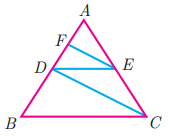Solution:
Given: In ∆ABC, DE || BC and CD || EF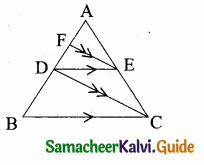To Prove: AD2 = AB × AF
Proof: In ∆ABC, DE || BC (Given)
By basic proportionality theorem
$$\frac { AB }{ AD }$$ = $$\frac { AC }{ AE }$$ ……….. (1)
In ∆ADC; FE || DC (Given)
By basic Proportionality theorem
$$\frac { AD }{ AF }$$ = $$\frac { AC }{ AE }$$ ……..(2)
From (1) and (2) we get
$$\frac { AB }{ AD }$$ = $$\frac { AD }{ AF }$$
Hence it is proved

Question 8.
In ∆ABC, AD is the bisector of ∠A meeting side BC at D, if AB = 10 cm, AC = 14 cm and BC = 6 cm, find BD and DC.
Solution:
In ∆AABC AD is the internal bisector of ∠A
Given BC = 6 cm
Let BD = x ∴ DC = 6 – x cm
By Angle bisector theorem
$$\frac { BD }{ DC }$$ = $$\frac { AB }{ AC }$$
$$\frac { x }{ 6-x }$$ = $$\frac { 10 }{ 14 }$$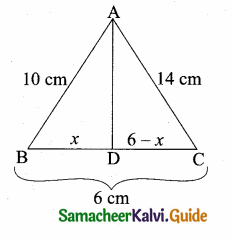14x = 60 – 10x
24x = 60
x = $$\frac { 60 }{ 24 }$$ = $$\frac { 10 }{ 4 }$$ = 2.5
BD = 2.5 cm;
DC = 6 – x ⇒ 2.5 = 3.5 cmQuestion 9.
Check whether AD is bisector of ∠A of ∆ABC in each of the following,
(i) AB = 5 cm, AC = 10 cm, BD = 1.5 cm and CD = 3.5 cm.
(ii) AB = 4 cm, AC 6 cm, BD = 1.6 cm and CD = 2.4 cm.
Solution:
(i) In ∆ABC, AB = 5 cm, AC = 10 cm, BD = 1.5 cm, CD = 3.5 cm
$$\frac { BD }{ DC }$$ = $$\frac { 1.5 }{ 3.5 }$$ = $$\frac { 15 }{ 35 }$$ = $$\frac { 3 }{ 7 }$$
$$\frac { AB }{ AC }$$ = $$\frac { 5 }{ 10 }$$ = $$\frac { 1 }{ 2 }$$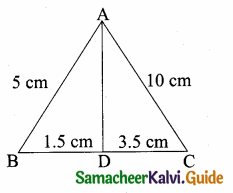$$\frac { BD }{ DC }$$ ≠ $$\frac { AB }{ AC }$$
∴ AD is not a bisector of ∠A.

(ii) In ∆ABC, AB = 4 cm, AC = 6 cm, BD = 1.6 cm, CD = 2.4 cm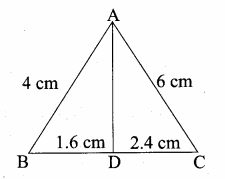$$\frac { BD }{ DC }$$ = $$\frac { 1.6 }{ 2.4 }$$ = $$\frac { 16 }{ 24 }$$ = $$\frac { 2 }{ 3 }$$
$$\frac { AB }{ AC }$$ = $$\frac { 4 }{ 6 }$$ = $$\frac { 2 }{ 3 }$$
∴ $$\frac { BD }{ DC }$$ = $$\frac { AB }{ AC }$$
By angle bisector theorem; AD is the internal bisector of ∠AQuestion 10.
In figure ∠QPR = 90°, PS is its bisector.
If ST ⊥ PR, prove that ST × (PQ + PR) = PQ × PR.
Solution:
Given: ∠QPR = 90°; PS is the bisector of ∠P. ST ⊥ ∠PR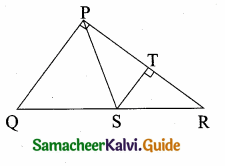To prove: ST × (PQ + PR) = PQ × PR
Proof: In ∆ PQR, PS is the bisector of ∠P.
∴ $$\frac { PQ }{ QR }$$ = $$\frac { QS }{ SR }$$
1 + $$\frac { PQ }{ QR }$$ = 1 + $$\frac { QS }{ SR }$$
$$\frac { PR+PQ }{ PR }$$ = $$\frac { SR+QS }{ SR }$$
$$\frac { PQ+PR }{ PR }$$ = $$\frac { QR }{ SR }$$ ……….(1)
In ∆ RST And ∆ RQP
∠SRT = ∠QRP = ∠R (Common)
∴ ∠QRP = ∠STR = 90°
(By AA similarity) ∆ RST ~ RQP
$$\frac { SR }{ QR }$$ = $$\frac { ST }{ PQ }$$
$$\frac { QR }{ SR }$$ = $$\frac { PQ }{ ST }$$ ……..(2)
From (1) and (2) we get
$$\frac { PQ+PR }{ PR }$$ = $$\frac { PQ }{ ST }$$
ST (PQ + PR) = PQ × PRQuestion 11.
ABCD is a quadrilateral in which AB = AD, the bisector of ∠BAC and ∠CAD intersect the sides BC and CD at the points E and F respectively. Prove that EF || BD.
Solution:
AE and AF are the internal bisector of ∠BAC and ∠DAC.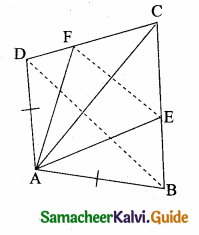To prove: EF || BD.
Construction: Join EF and BD
Proof: In ∆ ABC, AE is the internal bisector of ∠BAC.
By Angle bisector theorem, we have,
∴ $$\frac { AB }{ AC }$$ = $$\frac { BE }{ EC }$$ ………(1)
In ∆ ADC, AF is the internal bisector of ∠DAC
By Angle bisector theorem, we have,
$$\frac { AD }{ AC }$$ = $$\frac { DF }{ FC }$$
∴ $$\frac { AB }{ AC }$$ = $$\frac { DF }{ FC }$$ (AB = AD given) ………(2)
From (1) and (2), we get,
$$\frac { BE }{ EC }$$ = $$\frac { DF }{ FC }$$
Hence in ∆ BCD,
BD || EF (by converse of BPT)

Question 12.
Construct a ∆PQR which the base PQ = 4.5 cm, R = 35° and the median from R to RG is 6 cm.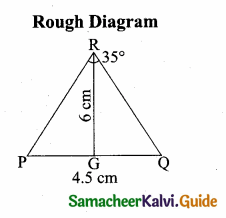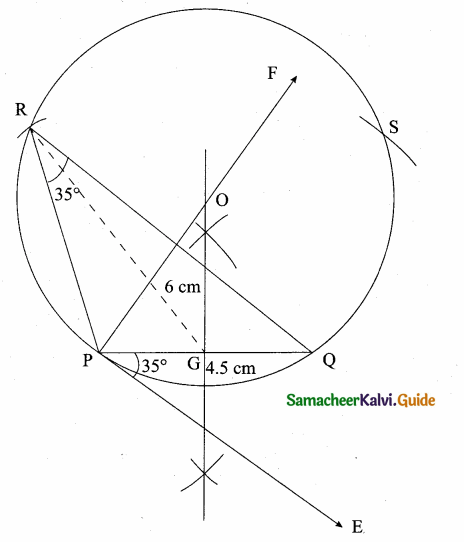Steps of construction

1. Draw a line segment PQ = 4.5 cm
2. At P, draw PE such that ∠QPE = 60°
3. At P, draw PF such that ∠EPF = 90°
4. Draw the perpendicular bisect to PQ, which intersects PF at O and PQ at G.
5. With O as centre and OP as radius draw a circle.
6. From G mark arcs of radius 5.8 cm on the circle. Mark them at R and S
7. Join PR and RQ. PQR is the required triangle.Question 13.
Construct a ∆PQR in which QR = 5 cm, ∠P = 40° and the median PG from P to QR is 4.4 cm. Find the length of the altitude from P to QR.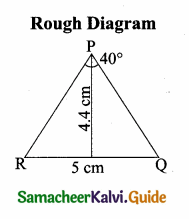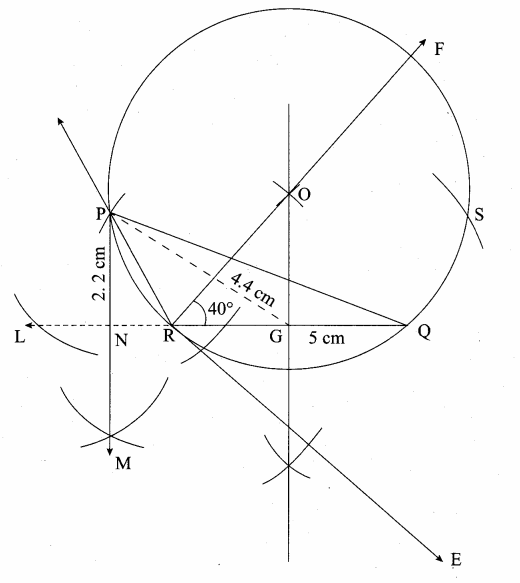Steps of construction

1. Draw a line segment RQ = 5 cm.
2. At R draw RE such that ∠QRE = 40°
3. At R, draw RF such that ∠ERF = 90°
4. Draw the perpendicular bisector to RQ, which intersects RF at O and RQ at G.
5. With O as centre and OP as radius draw a circle.
6. From G mark arcs of radius 4.4 cm on the circle. Mark them as P and S.
7. Join PR and PQ. Then ∆PQR is the required triangle.
8. From P draw a line PN which is perpendicular to RQ it meets at N.
9. Measure the altitude PN.
PN = 2.2 cm.Question 14.
Construct a ∆PQR such that QR = 6.5 cm, ∠P = 60° and the altitude from P to QR is of length 4.5 cm.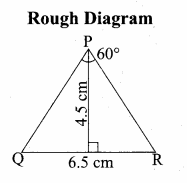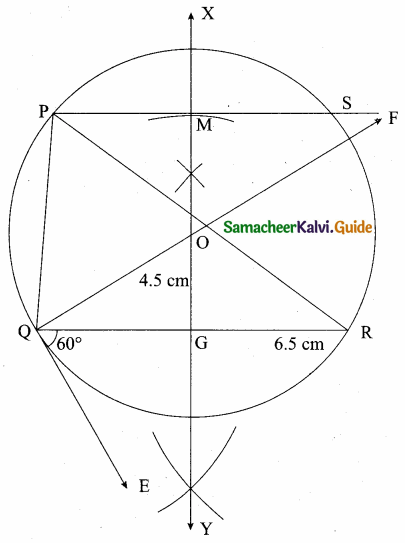Steps of construction

1. Draw a line segment QR = 6.5 cm.
2. At Q draw QE such that ∠RQE = 60°.
3. At Q, draw QF such that ∠EQF = 90°.
4. Draw the perpendicular of QR which intersects QF at O and QR at G.
5. With O as centre and OQ as radius draw a circle.
6. X Y intersects QR at G. On X Y, from G mark an arc at M. Such that GM = 4.5 cm.
7. Draw AB through M which is parallel to QR.
8. AB Meets the circle at P and S.
9. join QP and RP.
PQR is the required triangle.Question 15.
Construct a ∆ABC such that AB = 5.5 cm, ∠C = 25° and the altitude from C to AB is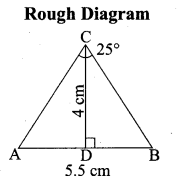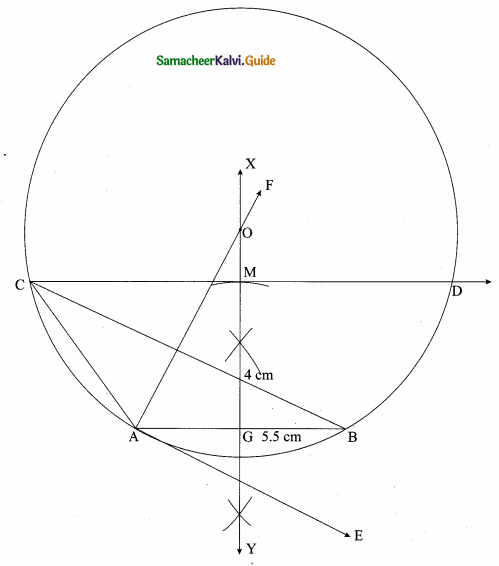Steps of construction

1. Draw a line segment AB = 5.5 cm.
2. At A draw AE such that ∠BAE = 25°.
3. At A draw AF such that ∠EAF = 90°.
4. Draw the perpendicular bisector of AB which intersects AF at O and AB at G.
5. With O as centre and OB as radius draw a circle.
6. X Y intersects AB at G. On X Y, from G mark an arc at M. Such that GM = 4 cm.
7. Through M draw a line parallel to AB intersect the circle at C and D.
8. Join AC and BC.
ABC is the required triangle.Question 16.
Draw a triangle ABC of base BC = 5.6 cm, ∠A = 40° and the bisector of ∠A meets BC at D such that CD = 4 cm.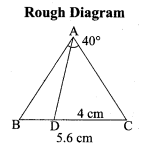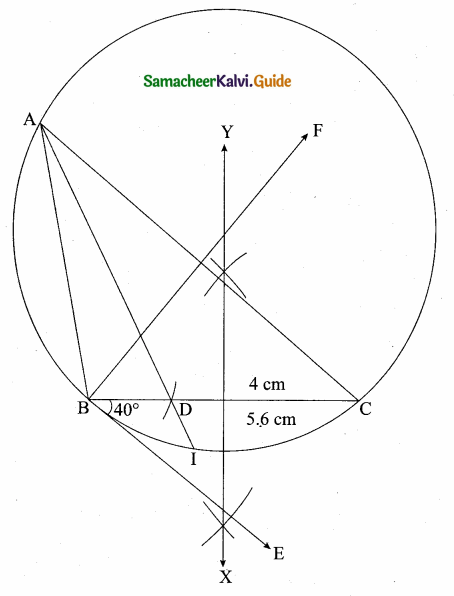Steps of construction

1. Draw a line segment BC = 5.6 cm.
2. At B draw BE such that ∠CBE = 40°.
3. At B draw BF such that ∠EBF = 90°.
4. Draw the perpendicular bisector to BC which intersects BF at O and BC at G.
5. With O as centre and OB as radius draw a circle.
6. From C mark an arc of 4 cm on CB at D.
7. The perpendicular bisector intersects the circle at I. Joint ID.
8. ID produced meets the circle at A. Now Join AB and AC.
This ABC is the required triangle.Question 17.
Draw ∆PQR such that PQ = 6.8 cm, vertical angle is 50° and the bisector of the vertical angle meets the base at D where PD = 5.2 cm